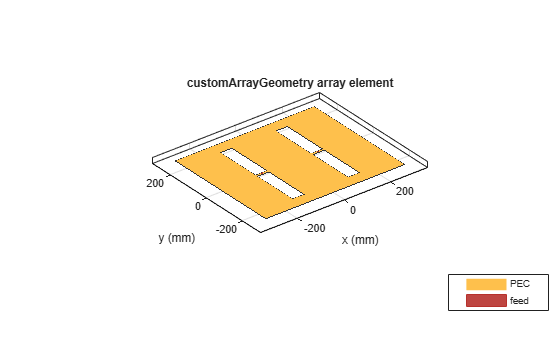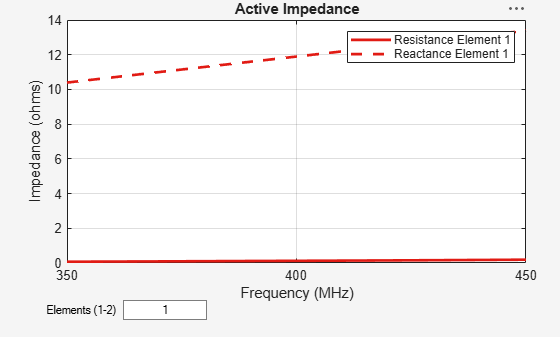# customArrayGeometry

Create array represented by 2-D custom geometry

## Description

The `customArrayGeometry` object is an array represented by a 2-D custom geometry on the X-Y plane. You can use the `customArrayGeometry` to import a 2-D custom geometry, define feeds to create an array element, and analyze the custom array.

## Creation

### Syntax

``array = customArrayGeometry``
``array = customArrayGeometry(Name,Value)``

### Description

``` `array = customArrayGeometry` creates a custom array represented by 2-D geometry on the X-Y plane, based on the specified boundary.```

example

``` `array = customArrayGeometry(Name,Value)` creates a 2-D array geometry, with additional properties specified by one or more name-value pair arguments. `Name` is the property name and `Value` is the corresponding value. You can specify several name-value pair arguments in any order as `Name1`, `Value1`, `...`, `NameN`, `ValueN`. Properties not specified retain their default values.```

### Output Arguments

expand all

Custom array geometry, returned as an `customArrayGeometry` object.

## Properties

expand all

Boundary information in Cartesian coordinates, specified as a cell array in meters.

Data Types: `double`

Boolean operation performed on the boundary list, specified as a character vector. operation set is; [`+`, `-`, `*`].

Example: `'Operation','P1-P2'`

Data Types: `double`

Array element feed location in Cartesian coordinates, specified as a three-element vector. The three elements represent the X, Y, and Z coordinates respectively.

Example: `'FeedLocation', [0 0.2 0]`

Data Types: `double`

Width of feed for array elements, specified as a scalar in meters.

Example: `'FeedWidth',0.05`

Data Types: `double`

Excitation amplitude for array elements, specified as a non-negative scalar or vector of non-negative scalars. Set property value to `0` to model dead elements.

Example: `'AmplitudeTaper',3`

Data Types: `double`

Phase shift for array elements, specified as a real scalar in degrees or a real vector in degrees.

Example: `'PhaseShift'`,[`3 3 0 0`] specified the phase shift for custom array containing four elements.

Data Types: `double`

Tilt angle of the array specified as a scalar or vector with each element unit in degrees. For more information, see Rotate Antennas and Arrays.

Example: `'Tilt',90`,

Example: `'Tilt',[90 90]`,`'TiltAxis',[0 1 0;0 1 1]` tilts the array at 90 degrees about the two axes, defined by vectors.

Data Types: `double`

Tilt axis of the array, specified as:

• Three-element vectors of Cartesian coordinates in meters. In this case, each vector starts at the origin and lies along the specified points on the X-, Y-, and Z-axes.

• Two points in space, each specified as three-element vectors of Cartesian coordinates. In this case, the array rotates around the line joining the two points in space.

• A string input describing simple rotations around one of the principal axes, 'X', 'Y', or 'Z'.

Example: `'TiltAxis',[0 1 0]`

Example: `'TiltAxis',[0 0 0;0 1 0]`

Example: `array.TiltAxis = 'Z'`

Data Types: `double`

## Object Functions

 `show` Display antenna or array structure; display shape as filled patch `info` Display information about antenna or array `axialRatio` Axial ratio of antenna `beamwidth` Beamwidth of antenna `charge` Charge distribution on metal or dielectric antenna or array surface `current` Current distribution on metal or dielectric antenna or array surface `EHfields` Electric and magnetic fields of antennas; Embedded electric and magnetic fields of antenna element in arrays `impedance` Input impedance of antenna; scan impedance of array `mesh` Mesh properties of metal or dielectric antenna or array structure `meshconfig` Change mesh mode of antenna structure `pattern` Radiation pattern and phase of antenna or array; Embedded pattern of antenna element in array `patternAzimuth` Azimuth pattern of antenna or array `patternElevation` Elevation pattern of antenna or array `returnLoss` Return loss of antenna; scan return loss of array `sparameters` Calculate S-parameter for antenna and antenna array objects `show` Display antenna or array structure; display shape as filled patch `vswr` Voltage standing wave ratio of antenna

## Examples

collapse all

Create a custom array using `customArrayGeometry`. Visualize it and plot the impedance. Also, visualize the current distribution on the array.

Create a ground plane with a length of 0.6 m and a width of 0.5 m.

```Lp = 0.6; Wp = 0.5; [~,p1] = em.internal.makeplate(Lp,Wp,2,'linear');```

Create slots on the ground plane with a length 0.05 m and a width of 0.4 m.

```Ls = 0.05; Ws = 0.4; offset = 0.12; [~,p2] = em.internal.makeplate(Ls,Ws,2,'linear'); p3 = em.internal.translateshape(p2, [offset, 0, 0]); p2 = em.internal.translateshape(p2, [-offset, 0, 0]);```

Create a feed in between the slots on the ground plane.

```Wf = 0.01; [~,p4] = em.internal.makeplate(Ls,Wf,2,'linear'); p5 = em.internal.translateshape(p4, [offset, 0, 0]); p4 = em.internal.translateshape(p4, [-offset, 0, 0]);```

Create an array using the slotted ground plane.

```carray = customArrayGeometry; carray.Boundary = {p1', p2', p3', p4', p5'}; carray.Operation= 'P1-P2-P3+P4+P5'; carray.NumFeeds = 2; carray.FeedWidth= [0.01 0.01]; carray.FeedLocation = [-offset,0,0 ; offset,0,0];```

Visualize the array.

`figure; show(carray);`Calculate the impedance of the array using the frequency range of 350 MHz to 450 MHz.

`figure; impedance(carray, 350e6:5e6:450e6);`Visualize the current distribution of the array at 410 MHz.

`figure; current(carray, 410e6);`Balanis, C. A. Antenna Theory. Analysis and Design. 3rd Ed. Hoboken, NJ: John Wiley & Sons, 2005.